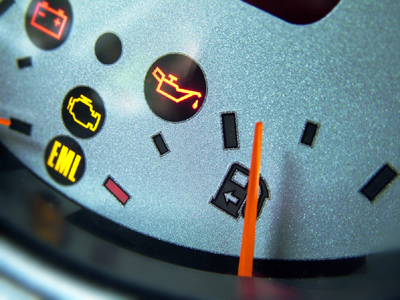For every 4 litres of petrol a car can travel 80km. 5 litres of petrol is required for a journey of 100km.

# Ratio (Year 6)

In KS2 Maths, you'll learn cool stuff about numbers and ratios. Imagine you have sand and water – in a ratio like 1:3. That means for every bit of sand, you need three bits of water. It's like a special way of comparing things!

Ratios are like magical tools that help us see how different things relate. It's super fun to play with numbers and make them dance together!

Want to test your ratio superpowers? Take the quiz and see if you can get a ratio of 10:0 right to wrong answers! Good luck, maths explorer!

1.
In every 12 tiles the proportion of blue tiles is 34. How many blue tiles in every 12?
3
6
8
9
34 of 12 is 9
2.
In a grid of 10 squares 4 are white and the rest are blue. What is the ratio of white to blue?
1:2
2:4
4:6
5:6
10 - 4 = 6 so there are 6 blue squares for every 4 white squares
3.
In every 10 tiles there are 2 red tiles. What is the proportion of red tiles?
13
14
15
12
210 and 2:10 are the same as 15 and 1:5
4.
For every 4 litres of petrol a car can travel 80km. How much petrol is required for a journey of 100km?
5 litres
8 litres
10 litres
12 litres
If 4 litres can travel 80km we divide by 4 to find 1 litre will travel 20km. So 5 litres will travel 100km
5.
If there is a 10:1 ratio between black squares and white squares which number represents white squares?
1
2
5
10
The 10 represents black and the 1 represents white because black was mentioned first and white second
6.
The proportion of brick houses is 310. How many brick houses in a street of 100 houses?
6
10
30
50
If 310 of the houses are brick, then 100 is 10 times 10 so we multiply by 10 to get 30100
7.
A biscuit recipe needs 250g flour, 150g of sugar and 150g of butter to make 6 biscuits. How much butter is needed to make 18 biscuits?
150g
300g
400g
450g
18 is 3 x 6 so we multiply 150g by 3
8.
In a box of chocolates there are 4 milk chocolates to 3 dark chocolates. How many dark chocolates in a box of 49 chocolates?
7
21
28
30
There are 3 dark chocolates in every 7. There are seven 7s in 49 so we multiply 3 by 7
9.
Jo mixes 400ml of orange juice to every 200ml of pineapple juice to make a fruit cocktail. How much orange juice will she need to make 900ml of fruit cocktail?
200ml
400ml
600ml
800ml
There is 400ml in every 600ml. 900ml = 1.5 x 600ml. So multiply 400ml by 1.5
10.
There are 2 white cubes for every 1 blue cube. What proportion are blue cubes to white cubes?
13
14
15
12
12 is the same as 1:2
You can find more about this topic by visiting BBC Bitesize - How to convert ratios into fractions and percentages

Author:  Amanda Swift